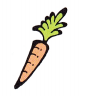# McGraw Hill Math Grade 3 Chapter 9 Lesson 7 Answer Key Estimating and Weighing in Ounces and Pounds

## McGraw-Hill Math Grade 3 Answer Key Chapter 9 Lesson 7 Estimating and Weighing in Ounces and Pounds

Estimate

Place a check mark beside the better estimate of each object’s weight.

Question 1.__________ 1 lb
__________ 1 oz
The estimated weight of the object is 1 lb.

Question 2.__________ 3 oz
__________ 3 lb
The estimated weight of the object is 3 oz.

Question 3.__________ 500 oz
__________ 155 lb
The estimated weight of the object is 155 lb.

Question 4.__________ 10 lb
__________ 14 oz
The estimated weight of the object is 14 oz.

Question 5.
Which weighs more: 16 ounces of feathers or 1 pound of marbles? Explain.